×#### Thank you for registering.

One of our academic counsellors will contact you within 1 working day.

Click to Chat

1800-1023-196

+91-120-4616500

CART 0

• 0

MY CART (5)

Use Coupon: CART20 and get 20% off on all online Study Material

ITEM
DETAILS
MRP
DISCOUNT
FINAL PRICE
Total Price: Rs.

There are no items in this cart.
Continue Shopping• Complete JEE Main/Advanced Course and Test Series
• OFFERED PRICE: Rs. 15,900
• View Details

```Chapter 1: Real Numbers Exercise – 1.5

Question: 1

Show that the following numbers are irrational.

(i) 7 √5

(ii) 6 + √2

(iii) 3 - √5

Solution:

(i) Let us assume that 7 √5 is rational. Then, there exist positive co primes a and b such that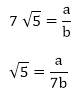We know that √5 is an irrational number

Here we see that √5 is a rational number which is a contradiction.

(ii) Let us assume that 6+√2 is rational. Then, there exist positive co primes a and b such that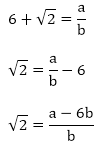Here we see that √2 is a rational number which is a contradiction as we know that √2 is an irrational number

Hence 6 + √2 is an irrational number

(iii) Let us assume that 3 - √5 is rational. Then, there exist positive co primes a and b such that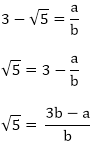Here we see that √5 is a rational number which is a contradiction as we know that √5 is an irrational number

Hence 3 - √5 is an irrational number.

Question: 2

Prove that the following numbers are irrationals.

(i) 2√7

(ii) 3/(2√5)

(iii) 4 + √2

(iv)  5√2

Solution:

(i) Let us assume that 2√7 is rational. Then, there exist positive co primes a and b such that√7 is rational number which is a contradiction

Hence 2√7 is an irrational number

(ii) Let us assume that 3/(2√5) is rational. Then, there exist positive co primes a and b such that√5 is rational number which is a contradiction

Hence 3/(2√5) is irrational.

(iii) Let us assume that 3/(2√5) is rational. Then, there exist positive co primes a and b such that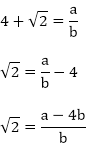√2 is rational number which is a contradiction

Hence 4+ √2 is irrational.

(iv) Let us assume that 5√2 is rational. Then, there exist positive co primes a and b such that√2 is rational number which is a contradiction

Hence 5√2 is irrational

Question: 3

Show that 2 - √3 is an irrational number.

Solution:

Let us assume that 2 - √3 is rational. Then, there exist positive co primes a and b such that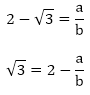Here we see that √3 is a rational number which is a contradiction

Hence 2- √3 is irrational

Question: 4

Show that 3 + √2 is an irrational number.

Solution:

Let us assume that 3 + √2 is rational. Then, there exist positive co primes a and b such thatHere we see that √2 is a irrational number which is a contradiction

Hence 3 + √2 is irrational

Question: 5

Prove that 4 - 5√2 is an irrational number.

Solution:

Let us assume that 4 - 5√2 is rational. Then, there exist positive co primes a and b such that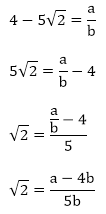This contradicts the fact that √2 is an irrational number

Hence 4 – 5√2 is irrational

Question: 6

Show that 5 - 2√3 is an irrational number.

Solution:

Let us assume that 5 -2√3 is rational. Then, there exist positive co primes a and b such thatThis contradicts the fact that √3 is an irrational number

Hence 5 – 2√3 is irrational

Question: 7

Prove that 2√3 - 1 is an irrational number.

Solution:

Let us assume that 2√3 – 1 is rational. Then, there exist positive co primes a and b such thatThis contradicts the fact that √3 is an irrational number

Hence 5 - 2√3 is irrational

Question: 8

Prove that 2 - 3√5 is an irrational number.

Solution:

Let us assume that 2 - 3√5 is rational. Then, there exist positive co primes a and b such that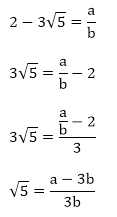This contradicts the fact that √5 is an irrational number

Hence 2 - 3√5 is irrational

Question: 9

Prove that √5 + √3 is irrational.

Solution:

Let us assume that √5 + √3 is rational. Then, there exist positive co primes a and b such that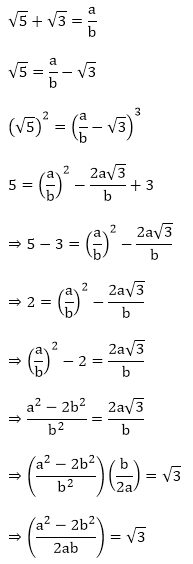Here we see that √3 is a rational number which is a contradiction as we know that √3 is an irrational number

Hence √5 + √3 is an irrational number

Question: 10

Prove that √3 + √4 is irrational.

Solution:

Let us assume that √3 + √4 is rational. Then, there exist positive co primes a and b such that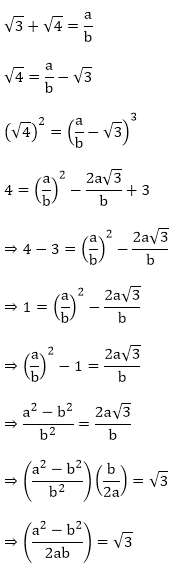Here we see that √3 is a rational number which is a contradiction as we know that √3 is an irrational number

Hence √3 + √4   is an irrational number

Question: 11

Prove that for any prime positive integer p, √p is an irrational number.

Solution:

Let us assume that √p is rational. Then, there exist positive co primes a and b such that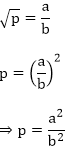⇒ pb2 = a2

⇒ p|a2 ⇒ p|a2

⇒ p|a ⇒ p|a

⇒ a = pc for some positive integer c ⇒ a = pc for some positive integer c

b2p = a2

⇒ b2p = p2c2 ( ∵ a = pc)

⇒ p|b2 (since p|c2p) ⇒ p|b2(since p|c2p)

⇒ p|b ⇒ p|a and p|b

This contradicts the fact that a and b are co primes

Hence √p is irrational

Question: 12

If p, q are prime positive integers, prove that √p + √q is an irrational number.

Solution:

Let us assume that √p + √q is rational. Then, there exist positive co primes a and b such thatHere we see that √q is a rational number which is a contradiction as we know that √q is an irrational number

Hence √p + √q  is an irrational number

```### Course Features

• 728 Video Lectures
• Revision Notes
• Previous Year Papers
• Mind Map
• Study Planner
• NCERT Solutions
• Discussion Forum
• Test paper with Video Solution